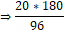Question of The Day07-03-2022

A and B can complete the work in 20 days. B is 20% less efficient then A. If C is 20% more efficient than B. Find the time taken by C to complete the work alone.

Correct Answer : a ) 37.5 days

Explanation :

According to the question

B is 20% less efficient than A

Let the efficiency of A be 100 units

⇒ B is 20% less efficient than A

⇒ efficiency of B = 100 – 20% of 100

⇒ 80 units

C is 20% more efficient than B

⇒ Efficiency of C = 80 + 20% of 80

⇒ 80 + 16

⇒ 96 units

We know that

Work = efficiency * (Number of days)

A and B can complete the work in 20 days

⇒ Work = (Efficiency of A and B) * 20

⇒ (100 + 80) * 20

⇒ 20 * 180 = 1600 units

Days taken by C to complete the work alone⇒ 37.5 days

Hence, (a) is the correct answer.

Attempt Time and Work Quiz for SSC CGL Tier 2.0